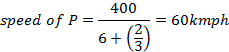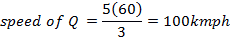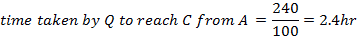## Ratio and Proportion For SBI PO : Set – 02

1) The ratio of the present age of A to that of B is 5 : 3. After 16 years the total of their ages will be 96 years. What will be the ratio of their ages at that time?

a) 5:4

b) 6:5

c) 7:4

d) 7:5

e) 8:5

(d)

A = 5x

B = 3x

After 16 years = (5x + 16) + (3x + 16) = 96

8x + 32 = 96

8x = 64

X = 8

Present ages of A & B = 40 & 24

After 16 years = 56: 40

= 7: 5

2) 16 liters are drawn from a cask full of wine and is then filled with water. This operation is performed three times. The ratio of the quantity of wine now left in cask to that of the water is 343:386. Now much wine the cask hold?

a) 34

b) 55

c) 62

d) 72

e) None of these

d)

X = ?; y = 16;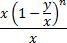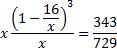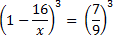x=72

3) Consider two alloys A and B  70 kg of alloy A is mixed with 140 kg of alloy b)   A contains brass and copper in the ratio 3 : 2, and B contains them in the ratio 4 : 3 respectively. What is the ratio of copper to brass in the mixture?

a) 84 : 52

b) 70:50

c) 50:12

d) 48:94

e) 44:61

e)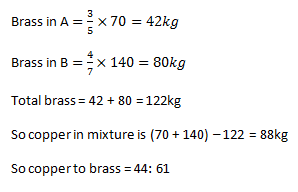4) The ratio of the present ages of a sankar and kumar is 1 : 5 and that of his friends maha and kumar is 4:5. After 2 years the ratio of the ages of the sankar to that of his friend maha becomes 3:10. What is the present age of the kumar?

a) 37 years

b) 35

c) 44

d) 39

e) None of these

b)

Let the present age of the sankar be x years

Present age of the kumar = 5x

Present age of the maha=4x

after 2 years,

age of the Sankar = x+2

age of the maha = 4x+2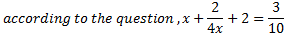10(x+2)=3(4x+2)

10x+20=12x+6

2x=14

5x=5 *7=35

2x=14

Present age of the Kumar = 35 years

5) There are 43800 students in 4 schools of a city. If half of the first , two-third of the second, three-fourth of the third and four-fifth of the fourth are the same number of students, then find the ratio of number of students of A and D if A, B, C and D be the first, second, third and fourth schools respectively.

a) 5:9

b) 7:6

c) 9: 7

d) 8: 5

e) 9: 5

Ans. D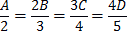A: B = 4 : 3

B: C = 9: 8

C: D = 16: 15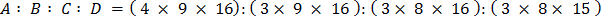A: D = 576: 360

A: D = 8: 5

6) Rs 900 were divided among A,B and C in such a way that A had Rs.60 more than B and C had Rs. 30 more than A. How much was C’s Share?

a) Rs 270

b) Rs 340

c) Rs.135

d) Rs.235

e) None of the above

b)

Let B have x Rs with him

Then A has = (60+x)

Then C has = (30+A) = (30+60+x) = 90+x

Hence A+B+C=900

60+x+x+90+x=900

3x+150=900

3x=750

x=250

Then C gets 90+x=90+250=340 Rs

7) Three friends Alice, Bond and Charlie divide \$1105 amongst them in such a way that if \$10, \$20 and \$15 are removed from the sums that Alice, Bond and Charlie received respectively, then the share of the sums that they got will be in the ratio of 11 : 18 : 24. How much did Charlie receive?

a) \$495

b) \$510

c) \$480

d) \$375

e) \$360

(a)

Let the sums of money received by A, B and C be x, y and z respectively.
Then x – 10 : y – 20 : z -15 is 11a : 18a : 24a
When \$10, \$20 and \$15 are removed, we are removing a total of \$45 from \$1105.
Therefore,

11a+18a+24a=1105-45=1060

53a=1060

or a= 20

We know that z – 15 = 24a = (24 * 20) = 480
Therefore, z = 480 + 15 = \$495

8) P, Q and R invest in a business. If the ratio of their time period are 3 : 4 : 5 and their profits are in the ratio 5 : 6 : 8. Find the ratio in which the investment are made by P, Q and R.

a) 50:45:48

b) 51:45:47

c) 51:46:48

d) 50 : 45 : 47

e) 50:47:48

a)

Ratio of their investment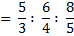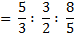50:45:48

9) A shopkeeper mixes two types of wheat, each costing Rs. 45/kg and Rs. 58/kg, so that by selling the resultant mixture at Rs. 55/kg, he makes a profit of 10%. In which ratio did he mix them?

a) 3:10

b) 1:1

c) 8:5

d) 2:7

e) None of these

c)

Using rule of allegation

Selling price = 55

Profit%=10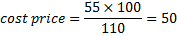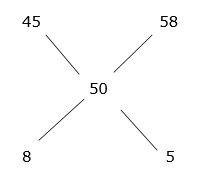Therefore the mixed ratio is 8:5

10) There are three persons Abi, Bala and Chan, not on the same straight road. Two buses P and Q start simultaneously from Abi and Bala respectively towards Chan. By the time Q reaches Chan, P is exactly halfway to Chan. Immediately after Q reaches Chan, it starts travelling towards Abi and  it crosses P at a point 165 km from Abi. The ratio of the speeds of P and Q is 3 : 5. Assume that the roads joining Abi to Chan, Bala to Chan and Bala to Abi  all are in straight roads. If Bala is twice as far as from Abi as it is from Chan and P would take to cover the distance from Abi to Bala, how much time would Q take to cover the distance from Chan to Abi?

a) 2.4hr

b) 3hr

c) 2.5hr

d) 5hr

e) None of these

a)

Let Abi = A

bala=B

chan= C

BC= 5k

given by the time Q reaches C, P was half way to C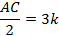and

AC=6k

as Q met P ,165 km away from A ,the distance to the meeting point from A is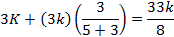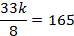k=40

AC=240km

BC=200km